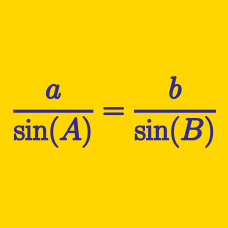Geometry

# Sine Rule - Ambiguous Case

How many distinct triangles are there such that $a=6, c=15, \angle A=30^\circ?$

Note: $a, b$ and $c$ are the lengths of the sides opposite the vertices $A,$ $B$ and $C,$ respectively.

How many distinct triangles are there such that $a=20, c=16, \angle A=30^\circ?$

Note: $a, b$ and $c$ are the lengths of the sides opposite the vertices $A,$ $B$ and $C,$ respectively.

How many distinct triangles are there such that $\angle A=30^\circ, a=9, b=9\sqrt{3} ?$

Note: $a, b$ and $c$ are the lengths of the sides opposite the vertices $A,$ $B$ and $C,$ respectively.

In triangle $ABC$, $a=13\sqrt{3}$, $b=13$, $\angle B=30^{\circ}$, and $\angle C$ is acute. What is the value of $\angle C$ (in degrees)?

Details and assumptions

$a$, $b$ and $c$ are the lengths of the sides opposite to the vertices $A$, $B$ and $C$, respectively. An acute angle is an angle strictly less than $90^\circ$.

How many distinct triangles are there such that $a=35, b=56, \angle A=30^\circ?$

Note: $a, b$ and $c$ are the lengths of the sides opposite the vertices $A,$ $B$ and $C,$ respectively.

×